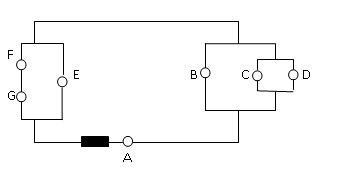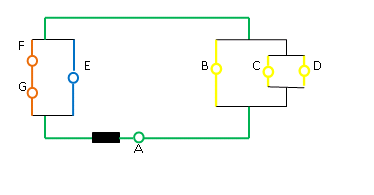# University of Wisconsin Green Bay

Rank the brightness of the identical light bulbs in the circuit below.• The brightness of a lightbulb is given by its power. P = I2R, and so brightness depends on current and resistance. If the bulbs are identical, they have the same resistance. They may not, however, experience the same current. Therefore, when you are asked to rank the brightness of identical bulbs, you are really being asked to rank the amount of current through each. This, then, is a circuit problem even though you do not need to specify the values of the currents.

•Because you do not need to solve this circuit mathematically, you do not need to reduce it to a single equivalent resistance. You do, however, need to track current. Using color to show portions of the circuit with the same current gives a very helpful visual.

• There are two ideas (charge is conserved, energy is conserved) and three equations that help you understand circuits.

As applied to circuits,

Conservation of Charge gives: Current is the same through resistors in series.

Conservation of Energy gives: Voltage drop is the same across each leg of a parallel circuit.

The three equations are

Req = ΣiRi Conceptually, this means that as more resistors are added in series the resistance of the circuit element increases.

1/R = Σi1/Ri Conceptually, this means that as more resistors are added in parallel the resistance of the circuit element decreases.

ΔV = IR Conceptually, this means that there is a "trade" between current and resistance in order to give the same voltage drop.

Because you are not asked for numbers, you only need to use the conceptual understandings behind these equations. If you think better mathematically, it is fine to use numbers to first see the relationships. Just make sure you then use that insight to understand the problem conceptually as well.

•Because all of the current passes through the green portions of the circuit, we know that Bulb A has the greatest amount of current and is therefore the brightest.

Each leg of the yellow portion of the circuit has the same resistance. In other words, a current element will flow through Bulb B or Bulb C or Bulb D, and, because the resistances are the same, the amount of current through each is the same. Therefore, Bulbs B, C, and D have the same current and, consequently, the same brightness. Furthermore, because the green current splits into three equal parts, the yellow current is 1/3 the value of the green current.

The current does not split evenly at this junction. Bulbs F and G are in series, and so the resistance of the orange leg is twice that of the blue leg. As a result, twice as much current goes through Bulb E as through Bulb F or G. Because the blue and orange currents add to the green current, the blue current is 2/3 the value of the green current, and the orange current is 1/3 the value of the green current.

Putting this together, A is the brightest bulb, followed by E. Bulbs B, C, D F and G are all the same brightness (and the faintest.)

•In this problem, you are asked to understand current flow in a circuit conceptually. You know that the current out through the battery splits and recombines at junctions and electrons are not used up. Furthermore, you know that current does not split evenly at a junction. Rather, more current passes through branches that have less resistance and IR always has the same value for branches in the same parallel elemenet.

This understanding is all that is required in order to determine what fraction of the current from the battery goes through each branch of a circuit. Note that you can also use these conceptual ideas to solve circuits mathematically. If you break down the circuit into its equivalent resistance (go to the Draw page of Circuit II) and use that to find the total current (the green current in this case), you can find the other currents based on their fraction of the green current.